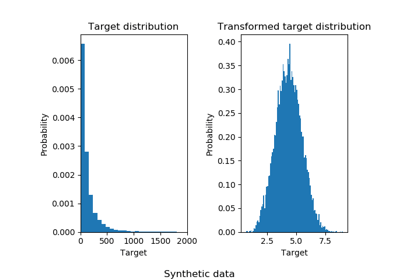/scikit-learn

# sklearn.compose.TransformedTargetRegressor

`class sklearn.compose.TransformedTargetRegressor(regressor=None, transformer=None, func=None, inverse_func=None, check_inverse=True)` [source]

Meta-estimator to regress on a transformed target.

Useful for applying a non-linear transformation in regression problems. This transformation can be given as a Transformer such as the QuantileTransformer or as a function and its inverse such as `log` and `exp`.

The computation during `fit` is:

```regressor.fit(X, func(y))
```

or:

```regressor.fit(X, transformer.transform(y))
```

The computation during `predict` is:

```inverse_func(regressor.predict(X))
```

or:

```transformer.inverse_transform(regressor.predict(X))
```

Read more in the User Guide.

Parameters: `regressor : object, default=LinearRegression()` Regressor object such as derived from `RegressorMixin`. This regressor will automatically be cloned each time prior to fitting. `transformer : object, default=None` Estimator object such as derived from `TransformerMixin`. Cannot be set at the same time as `func` and `inverse_func`. If `transformer` is `None` as well as `func` and `inverse_func`, the transformer will be an identity transformer. Note that the transformer will be cloned during fitting. Also, the transformer is restricting `y` to be a numpy array. `func : function, optional` Function to apply to `y` before passing to `fit`. Cannot be set at the same time as `transformer`. The function needs to return a 2-dimensional array. If `func` is `None`, the function used will be the identity function. `inverse_func : function, optional` Function to apply to the prediction of the regressor. Cannot be set at the same time as `transformer` as well. The function needs to return a 2-dimensional array. The inverse function is used to return predictions to the same space of the original training labels. `check_inverse : bool, default=True` Whether to check that `transform` followed by `inverse_transform` or `func` followed by `inverse_func` leads to the original targets. `regressor_ : object` Fitted regressor. `transformer_ : object` Transformer used in `fit` and `predict`.

#### Notes

Internally, the target `y` is always converted into a 2-dimensional array to be used by scikit-learn transformers. At the time of prediction, the output will be reshaped to a have the same number of dimensions as `y`.

#### Examples

```>>> import numpy as np
>>> from sklearn.linear_model import LinearRegression
>>> from sklearn.compose import TransformedTargetRegressor
>>> tt = TransformedTargetRegressor(regressor=LinearRegression(),
...                                 func=np.log, inverse_func=np.exp)
>>> X = np.arange(4).reshape(-1, 1)
>>> y = np.exp(2 * X).ravel()
>>> tt.fit(X, y)
TransformedTargetRegressor(...)
>>> tt.score(X, y)
1.0
>>> tt.regressor_.coef_
array([2.])
```

#### Methods

 `fit`(X, y[, sample_weight]) Fit the model according to the given training data. `get_params`([deep]) Get parameters for this estimator. `predict`(X) Predict using the base regressor, applying inverse. `score`(X, y[, sample_weight]) Returns the coefficient of determination R^2 of the prediction. `set_params`(**params) Set the parameters of this estimator.
`__init__(regressor=None, transformer=None, func=None, inverse_func=None, check_inverse=True)` [source]
`fit(X, y, sample_weight=None)` [source]

Fit the model according to the given training data.

Parameters: `X : {array-like, sparse matrix}, shape (n_samples, n_features)` Training vector, where n_samples is the number of samples and n_features is the number of features. `y : array-like, shape (n_samples,)` Target values. `sample_weight : array-like, shape (n_samples,) optional` Array of weights that are assigned to individual samples. If not provided, then each sample is given unit weight. `self : object`
`get_params(deep=True)` [source]

Get parameters for this estimator.

Parameters: `deep : boolean, optional` If True, will return the parameters for this estimator and contained subobjects that are estimators. `params : mapping of string to any` Parameter names mapped to their values.
`predict(X)` [source]

Predict using the base regressor, applying inverse.

The regressor is used to predict and the `inverse_func` or `inverse_transform` is applied before returning the prediction.

Parameters: `X : {array-like, sparse matrix}, shape = (n_samples, n_features)` Samples. `y_hat : array, shape = (n_samples,)` Predicted values.
`score(X, y, sample_weight=None)` [source]

Returns the coefficient of determination R^2 of the prediction.

The coefficient R^2 is defined as (1 - u/v), where u is the residual sum of squares ((y_true - y_pred) ** 2).sum() and v is the total sum of squares ((y_true - y_true.mean()) ** 2).sum(). The best possible score is 1.0 and it can be negative (because the model can be arbitrarily worse). A constant model that always predicts the expected value of y, disregarding the input features, would get a R^2 score of 0.0.

Parameters: `X : array-like, shape = (n_samples, n_features)` Test samples. For some estimators this may be a precomputed kernel matrix instead, shape = (n_samples, n_samples_fitted], where n_samples_fitted is the number of samples used in the fitting for the estimator. `y : array-like, shape = (n_samples) or (n_samples, n_outputs)` True values for X. `sample_weight : array-like, shape = [n_samples], optional` Sample weights. `score : float` R^2 of self.predict(X) wrt. y.
`set_params(**params)` [source]

Set the parameters of this estimator.

The method works on simple estimators as well as on nested objects (such as pipelines). The latter have parameters of the form `<component>__<parameter>` so that it’s possible to update each component of a nested object.

Returns: self

## Examples using `sklearn.compose.TransformedTargetRegressor`Effect of transforming the targets in regression model